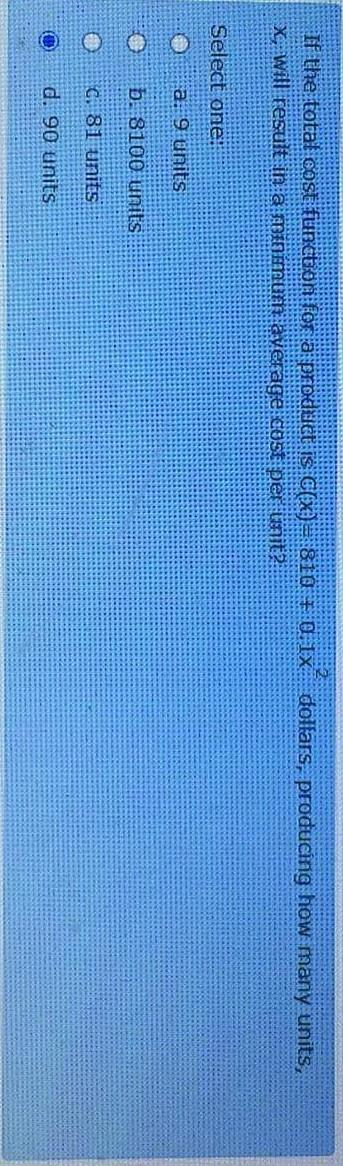### Create an Account

Home / Questions / If the total cost function for a prodüct is CEx)3 810 0.1X dollars, producing how many uni...

# If the total cost function for a prodüct is CEx)3 810 0.1X dollars, producing how many units, X will result in a minimum average cost per unit Select one: a: 9 Units b. 8100 units O C. 81 units d. 90

If the total cost function for a prodüct is CEx)3 810 0.1X dollars, producing how many units, X will result in a minimum average cost per unit Select one: a: 9 Units b. 8100 units O C. 81 units d. 90 unitsMay 30 2021 View more View LessSubscribe To Get Solution